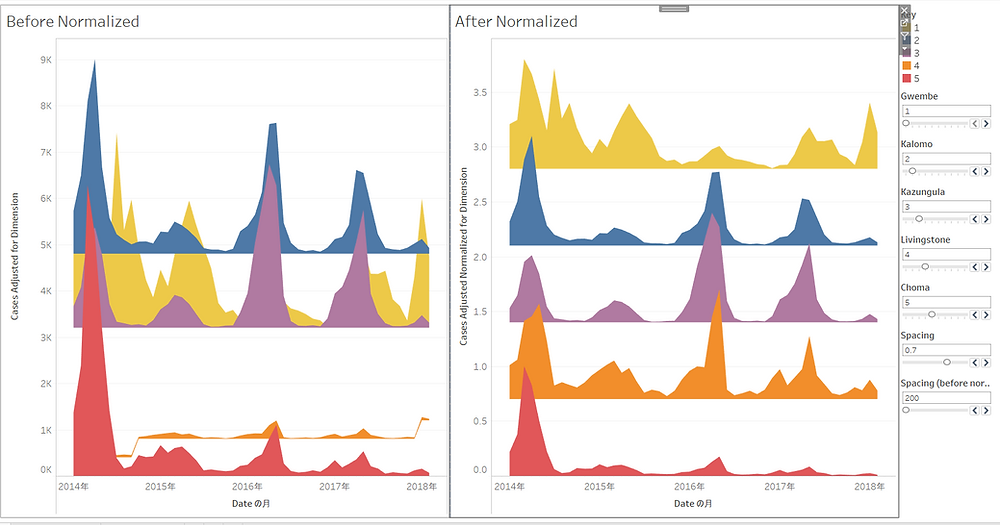At the previous post I introduced the Joy Plot, and I tried to use it with the #makeovermonday Week 16.

Then I faced one problem there. In this post I'd like to share the problem and the solution for it.

Let me introduced the data I used first. That was about Malaria cases in Zambia available here.

I used districts for the dimension of Joy Plot and reported cases for Value.

Then, I got this.Oops. The polygons has totally different scales and it's totally overlapping or totally separated.

In fact, the sum of cases by year and district is much different. It's almost 100 times different.So basically I think the problem here is the scale of the values of each dimension is different a lot.

Fortunately there's a popular method to scale a measure into 0 to 1. Here's the equation.For example, if you have data of [1, 2, 4, 6, 8] where the maximum is 8 and the minimum is 1,

Then the second data 2 can be normalized to (2 - 1) / (8 - 1) = 0.14. What if x = 8? (8 - 1) / (8 - 1) = 1.

Surely if x = 1 then x_normalized is 0.

Then, we will have another question: How can we do this in Tableau?

The solution is quite simple: by using WINDOW_MIN() and WINDOW_MAX().

So let's see the calculation. Like the ordinal joy plot, we need the adjusted value first.

CASE [Purpose] WHEN "Primary" THEN [Cases] ELSE 0 END

Then we use the window function.

Then we calculate the adjusted value for dimensions.

[Cases Adjusted Normalized] + ATTR({FIXED:COUNTD([District])} - [Key])*[Spacing]

Notice that since the Normalized calculation is aggregated, we also need to aggregate the later part by using ATTR(). As you may know, in this case ATTR() is almost for nothing except for the sake of making tableau recognize the later part aggregated.

And since the data was monthly data, I changed the PATH calculation a bit.

PATH

IF [Purpose]="Primary" THEN [Date] ELSE DATEADD('month',1,DATE((INT({FIXED [District]: MAX([Date])})*2) - INT([Date]))) END

And the result is here.In conclusion, if you want to create the Joy Plot with data that have different scales, please try the normalization.

My dashboard for this post is available here:

https://public.tableau.com/profile/yoshitaka6076#!/vizhome/JoyPlotwithNormalizationquitesimple/Sheet1?publish=yes

Also my new viz for #makeovermonday Week 16 is here.

https://public.tableau.com/profile/yoshitaka6076#!/vizhome/MakeoverMondayW16ZambiaMalariaCases-Redesigned/Dashboard1I hope you enjoyed this post. See you soon.

Yoshi

#Tableau